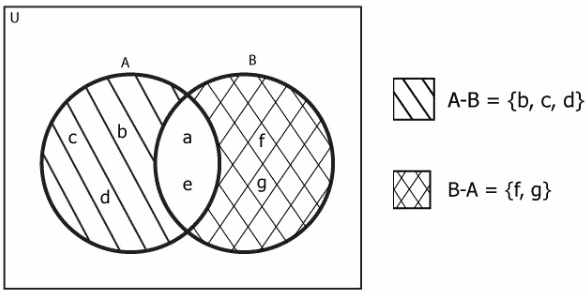# Python Set difference_update: Complete Guide

Python difference_update() method helps differentiate the set in the in-place. For example, the previously discussed Python set difference() helps determine the difference between two sets. It returns the new set with a different value, but the difference_update() updates the existing caller set.

For example, if  A = {a,b,c,d,e,f} and B={a,e,f,g} are two sets and if we want to find A-B then the result will be {b,c,d}, also this method will modify the set A with values {b,c,d}.Similarly, if we want to find B-A, the result will be {f,g}, and this method will modify the set B with this value but set A will remain unchanged.

## Python Set difference_update

Python Set difference_update() is a built-in method that finds the difference between two sets; also, it modifies the caller set with the result set. In the set difference method, we see the difference between the two sets and return the difference as a new set.

### Syntax

```First_Set.difference_update(Second_Set)
```

The above syntax will help us to find the difference between First_Set-Second_Set.

So, If we want to find A-B, the syntax will be:

```A.difference_update(B)
```

### Return Value

The function does not return any value, and it returns None. But it changes the value of the caller set.

See the following code example.

```# app.py

# Declaring two sets
A = {'a', 'b', 'c', 'd', 'e'}
B = {'a', 'e', 'f', 'g'}

# Now we will apply difference_update() to find A-B
result = A.difference_update(B)

# Now we will check the value of A, B, and result
print("Value of A is: ", A)
print("Value of B is: ", B)
print("Value of result is: ", result)
```

#### Output

```Value of A is:  {'c', 'b', 'd'}
Value of B is:  {'f', 'e', 'a', 'g'}
Value of result is:  None
```

From the above example, we can see that we could find the difference between sets A and B, but the method modified the value of set A with the value of A-B.

Also, B remains unchanged, and the value of the result is None, as the function does not return anything.